International
Tables for
Crystallography
Volume H
Powder diffraction
Edited by C. J. Gilmore, J. A. Kaduk and H. Schenk

International Tables for Crystallography (2018). Vol. H, ch. 1.1, pp. 9-11

Section 1.1.3. The peak intensity

R. E. Dinnebiera* and S. J. L. Billingeb,c

aMax-Planck-Institute for Solid State Research, Heisenbergstrasse 1, D-70569 Stuttgart, Germany,bDepartment of Applied Physics and Applied Mathematics, Columbia University, 500 West 120th Street, Room 200 Mudd, MC 4701, New York, NY 10027, USA, and cCondensed Matter Physics and Materials Science Department, Brookhaven National Laboratory, PO Box 5000, Upton, NY 11973–5000, USA
Correspondence e-mail:  r.dinnebier@fkf.mpg.de

1.1.3. The peak intensity

| top | pdf |

| top | pdf |

Bragg's law gives the positions at which diffraction by a crystal will lead to sharp peaks (known as Bragg peaks) in diffracted intensity. We now want to investigate the factors that determine the intensities of these peaks.

X-rays are electromagnetic (EM) waves with a much shorter wavelength than visible light, typically of the order of 1 Å (= 10−10 m). The physics of EM waves is well understood and excellent introductions to the subject are found in every textbook on optics. Here we briefly review the results that are most important in understanding the intensities of Bragg peaks.

Classical EM waves can be described by a sine wave of wavelength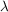that repeats every 2π radians. If two identical waves are not coincident, they are said to have a phase shift, which is either measured as a shift, Δ, on a length scale in units of the wavelength, or equivalently as a shift in the phase,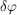, on an angular scale, such that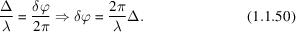This is shown in Fig. 1.1.14.Figure 1.1.14 | top | pdf |Graphical illustration of the phase shift between two sine waves of equal amplitude. [Reproduced from Dinnebier & Billinge (2008) with permission from the Royal Society of Chemistry.]

The detected intensity, I, is proportional to the square of the amplitude, A, of the sine wave. With two waves present that are coherent and can interfere, the amplitude of the resultant wave is not just the sum of the individual amplitudes, but depends on the phase shift. The two extremes occur when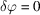(constructive interference), where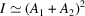, and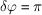(destructive interference), where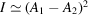. In general,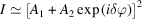. When more than two waves are present, this equation becomes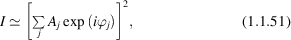where the sum is over all the sine waves present and the phases,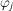, are measured with respect to some origin.

Measuring X-ray diffraction involves the measurement of the intensity of X-rays scattered from electrons bound to atoms. Waves scattered by atoms at different positions arrive at the detector with a relative phase shift. Therefore, the measured intensities yield information about the relative atomic positions.

In the case of X-ray diffraction, the Fraunhofer approximation is valid. This is a far-field approximation, where the distances L1 from the source to the place where scattering occurs (the sample) and L2 from the sample to the detector are much larger than the separation, D, of the scatterers. This is an excellent approximation, since in this case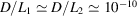. The Fraunhofer approximation greatly simplifies the mathematics. The incident X-rays come from a distant source and form a wavefront of constant phase that is a plane wave. X-rays scattered by single electrons are outgoing spherical waves, which again appear as plane waves in the far field. This allows us to express the intensity of the diffracted X-rays using equations (1.1.51)and (1.1.39).

This is the origin of equation (1.1.39), which gives the amplitude of the scattered radiation in terms of the scattering vector, h = s0s, and the atomic positions, rj. In fact, the amplitude of the scattered radiation is only proportional to this expression. The actual intensity depends on the amplitude of the incident wave and also on the absolute scattering power of the scatterers. If we neglect for now the incident intensity and assume that our measured intensities are normalized to the incident beam intensity, we get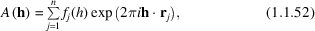where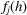is the atomic form factor and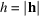is the magnitude of the scattering vector, and is described in more detail in International Tables for Crystallography, Volume C, Part 6. This is a measure of the strength of scattering from the jth atom. At, scattering is in the forward direction with all electrons scattering in phase. As a result, fj(0) equals the number of electrons bound to the atom (in units of the Thomson scattering cross section for an electron), usually taken to be the atomic number of the atomic species at the jth site. An additional h-dependent reduction of the amplitude comes from positional disorder of the atoms. A Gaussian blurring is used with a width that is often falsely called the temperature factor', but is more correctly known as the atomic displacement parameter (ADP). The Gaussian is known as the Debye–Waller factor, which is discussed below. More information can be found in Chapter 4.7.

The crystal structure consists of periodic arrangements of atoms. The simplest structures have one atom in a periodically repeated unit cell. However, in general, there is a well defined group of atoms that forms a structural motif that is periodically repeated. This motif can range from one atom to thousands of atoms in complex protein structures. Solving the crystal structure consists of finding the unit-cell parameters and determining the positions in the unit cell of the atoms in the structural motif. In this sense, the structure of the infinite crystal can be thought of mathematically as a convolution of the periodic lattice that we discussed above with the structural motif. This results in a perfect, orientationally ordered copy of the structural motif in every unit cell translated in three-dimensional space.

As we discussed above, the direct-space lattice has a reciprocal lattice associated with it which determines the positions of the Bragg peaks, or allowed delta functions of scattered intensity. The reciprocal lattice is actually a Fourier transform of the periodic lattice in direct space. The convolution theorem of Fourier transforms tells us that a convolution of two functions in direct space will result in a product of the Fourier transforms of those functions in the Fourier space. Since the structure is a convolution of the direct-space lattice with the structural motif, the reciprocal lattice will be multiplied by the Fourier transform of the structural motif. This Fourier transform of the structural motif is called the crystallographic structure factor, Fhkl.

This result can be readily derived from equation (1.1.52). In this equation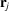is the vector from the (arbitrary but fixed) origin to the jth atom in the material. If we now think of the crystal as consisting of n identical cells, each containing an identical structural motif consisting of m atoms, we can writeas a sum of two vectors: a vector that goes from the origin to the corner of the sth unit cell that contains the jth atom, and a second vector that goes from the corner of the sth cell to the position of the jth atom. This is illustrated in Fig. 1.1.15.Figure 1.1.15 | top | pdf |The position vector of the jth atom rj can be decomposed into a vector Rs from the origin of the crystal to the origin of the unit cell containing the jth atom, and the vector ut from the unit cell origin to the jth atom.

Equation (1.1.52) can then be written as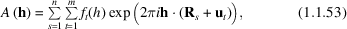where it is readily seen that the first sum is taken over all the cells in the crystal and the second sum is taken over the m atoms in the structural motif. The equation is readily factored as follows: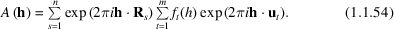Taking n to infinity, we immediately recognise the first sum as the lattice sum of equation (1.1.43), and we can therefore rewrite equation (1.1.54) as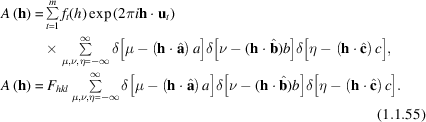The delta functions determine the positions of the reciprocal-lattice points (directions of the Bragg peaks), and their intensities are multiplied by a factor, the crystallographic structure factor,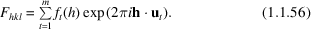If we write each term as a complex number denoted ft, we can represent this complex sum as a vector sum in the complex plane, as illustrated in Fig. 1.1.16, where the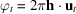. The intensity of the Bragg peak depends only on the length of the Fhkl, not its direction. However, its length depends on both the lengths and the phases of each contribution, which in turn depend on the positions of the atoms within the unit cell. This is the phase information that is lost' in a diffraction experiment. Given a structure, we can directly calculate all the Bragg-peak intensities (the forward problem'). However, given all the Bragg-peak intensities, we cannot directly calculate the structure (the inverse problem'). Structure determination uses the measured intensities and reconstructs the lost phase information using various iterative methods and algorithms.Figure 1.1.16 | top | pdf |Graphical illustration of the summation of scattered wave amplitudes ft in the complex plane, accounting for the phase shifts coming from the different positions of the atoms in the unit cell.

In fact, the intensity of a Bragg reflection hkl is given by the squared absolute value of the structure-factor amplitude Fhkl,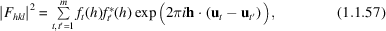where * indicates the complex conjugate. This analysis shows that the positions of the Bragg peaks determine the geometry of the periodic lattice (the size and shape of the unit cell, for example), but the intensities of the Bragg peaks are determined by the relative positions of atoms within the unit cell, scaled by their respective scattering power. To solve the internal structure of the structural motif within the unit cell, it is necessary to measure quantitatively the intensities of many Bragg peaks and use some kind of iterative procedure to move the atoms within the cell until the calculated structure factors self-consistently reproduce the intensities of all the measured Bragg peaks.

The situation is not fundamentally different in a powder diffraction experiment from the single-crystal case, except that the Bragg peaks in three-dimensional reciprocal space are projected into one dimension, as shown in Fig. 1.1.17.Figure 1.1.17 | top | pdf |Schematic illustration of the projection of the reciprocal a*c* plane (representing the three-dimensional reciprocal-lattice space) into the one-dimensional powder pattern.

`Indexing' is the term used for deriving the lattice parameters from the positions of the Bragg peaks (see Chapter 3.4). Once the size and shape of the reciprocal lattice is determined, Miller indices can be assigned to each of the Bragg peaks in a one-dimensional powder pattern. If it is possible to extract the intensities of those peaks from the pattern, diffraction data from a powder can be used to reconstruct the three-dimensional structure in exactly the same way as is done with data from a single crystal. This process is known as structure solution from powder diffraction, and is often successful, although it is less well automated than structure solution from data from single crystals. As mentioned above, the main problem with powder data is a loss of information due to systematic and accidental peak overlap, but this can often be overcome.

There are various methods for extracting quantitative peak intensities from indexed powder patterns by computer fitting of profiles to the Bragg peaks at their known positions. Two of the most common are Pawley refinement (Pawley, 1981) and Le Bail refinement (Le Bail et al., 1988), as discussed in Chapter 3.5.

In general, the intensities of the Bragg reflections must be corrected by the product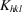of various correction factors. Some common correction factors are given by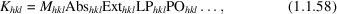where Mhkl is the multiplicity, Abshkl is an absorption correction, Exthkl is an extinction correction, LPhkl is the geometrical Lorentz–polarization correction and POhkl is a correction for preferred orientation (see Chapter 4.7).

If there is more than one crystalline phase present in the sample, and the structures of all the crystalline phases are known, then we can find a scale factor for each phase in the mixture which reproduces the data. This is then a way of determining the proportion of each phase in the sample. This is called quantitative phase analysis (see Chapter 3.9).

References

Le Bail, A., Duroy, H. & Fourquet, J. L. (1988). Ab-initio structure determination of LiSbWO6 by X-ray powder diffraction. Mater. Res. Bull. 23, 447–452.Google Scholar
Pawley, G. S. (1981). Unit-cell refinement from powder diffraction scans. J. Appl. Cryst. 14, 357–361.Google Scholar